# law_of_sines.ppt

1. Jun 2023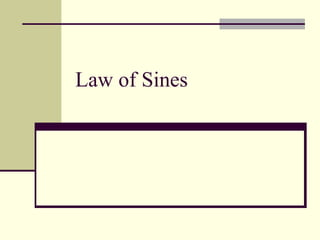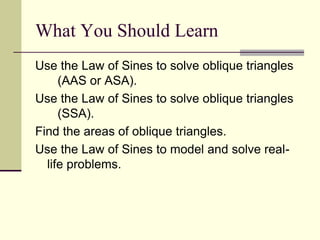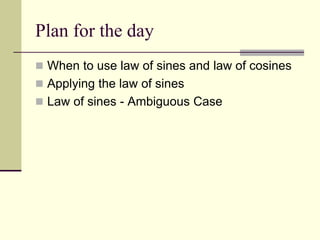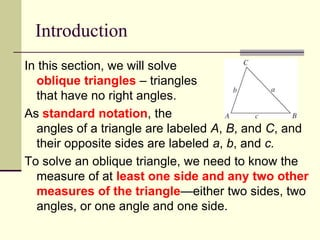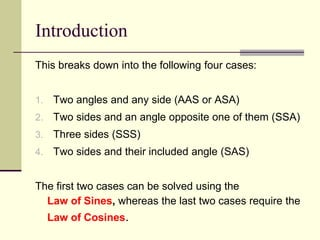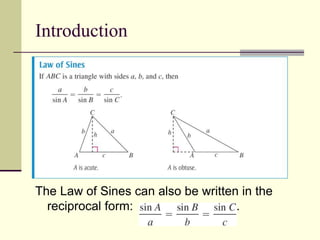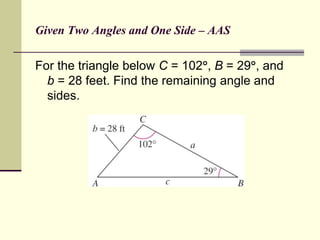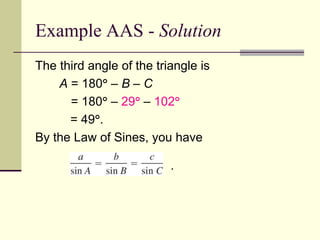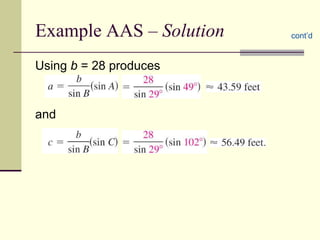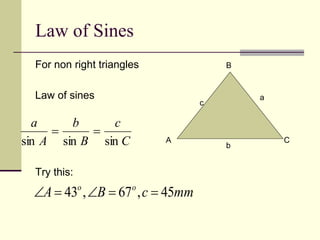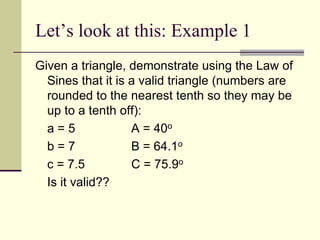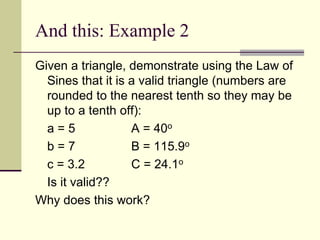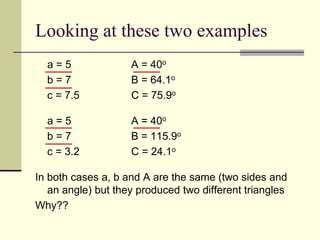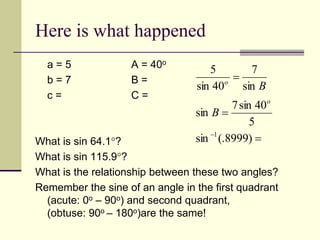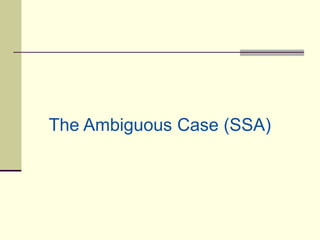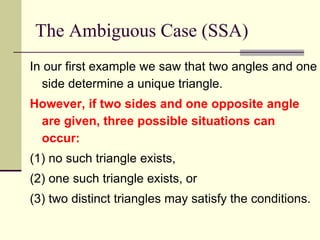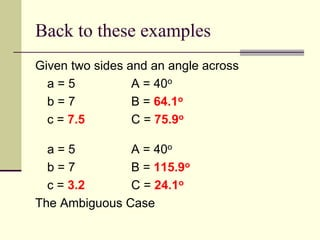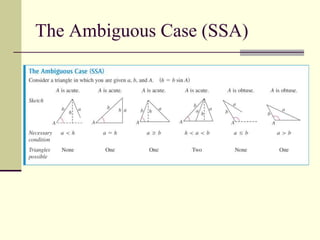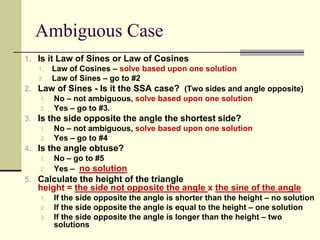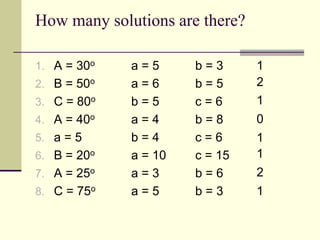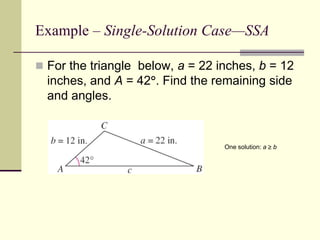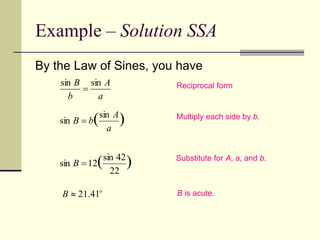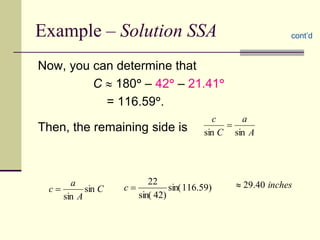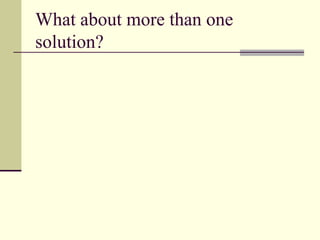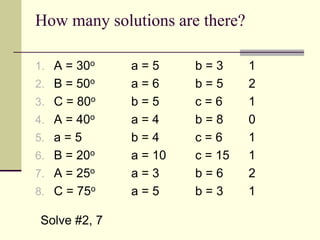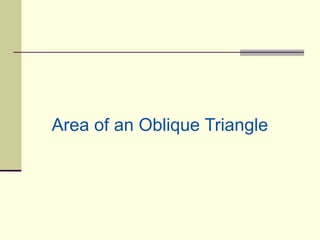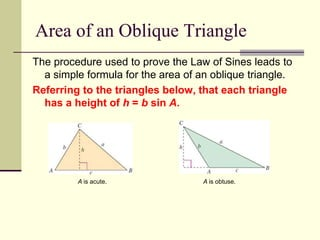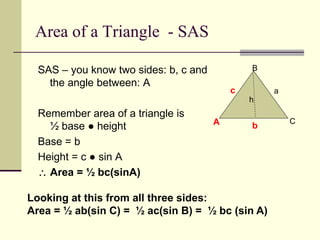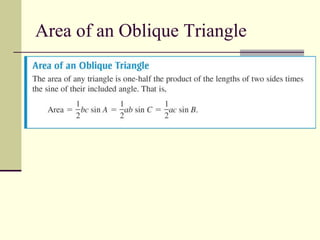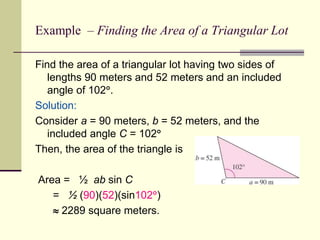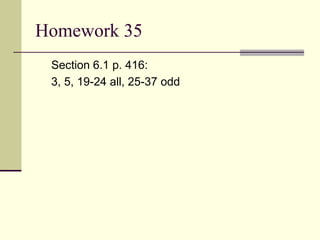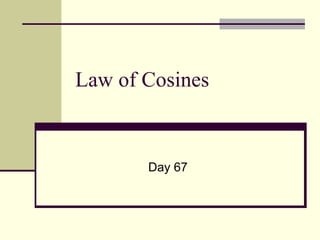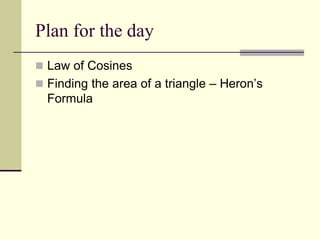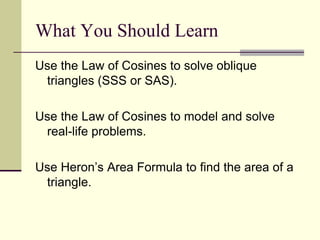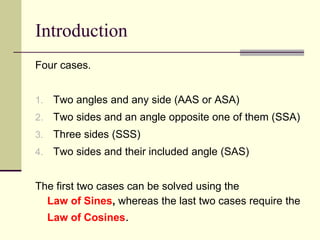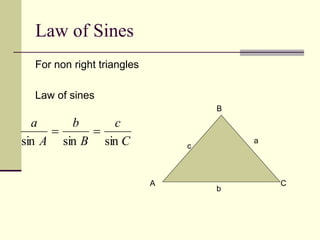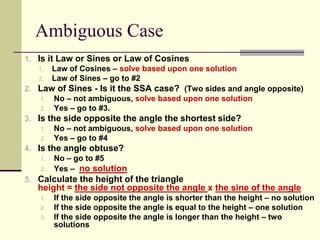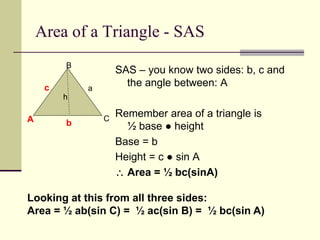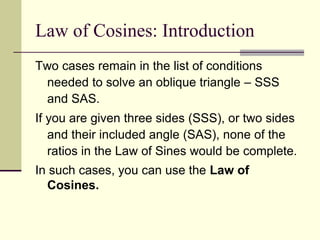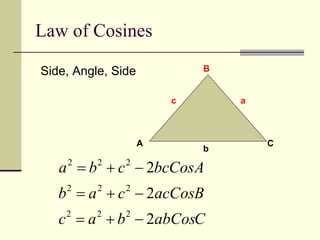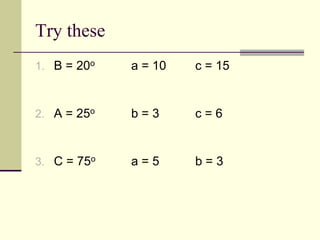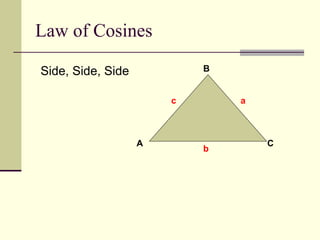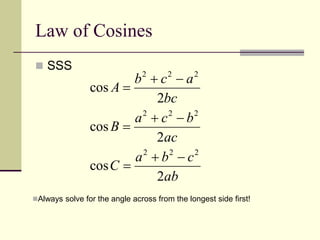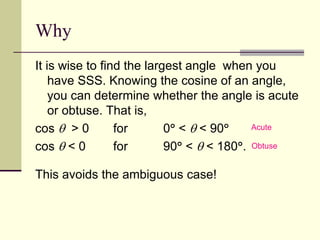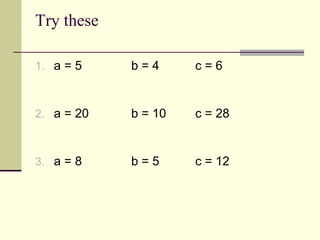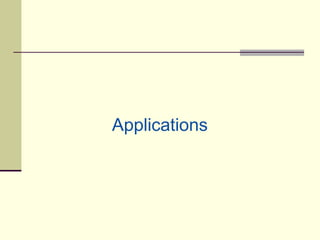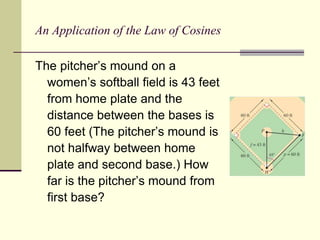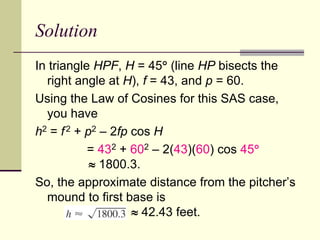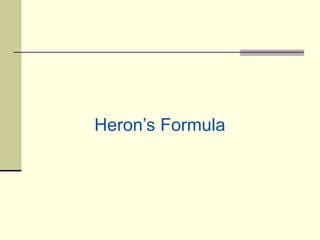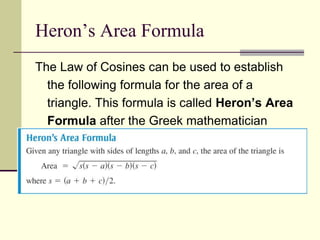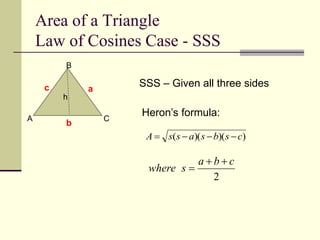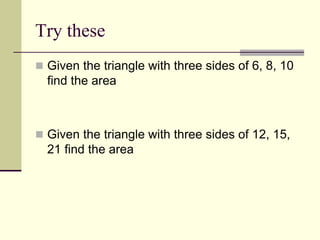1 von 52

### law_of_sines.ppt

• 2. Use the Law of Sines to solve oblique triangles (AAS or ASA). Use the Law of Sines to solve oblique triangles (SSA). Find the areas of oblique triangles. Use the Law of Sines to model and solve real- life problems. What You Should Learn
• 3. Plan for the day  When to use law of sines and law of cosines  Applying the law of sines  Law of sines - Ambiguous Case
• 4. Introduction In this section, we will solve oblique triangles – triangles that have no right angles. As standard notation, the angles of a triangle are labeled A, B, and C, and their opposite sides are labeled a, b, and c. To solve an oblique triangle, we need to know the measure of at least one side and any two other measures of the triangle—either two sides, two angles, or one angle and one side.
• 5. Introduction This breaks down into the following four cases: 1. Two angles and any side (AAS or ASA) 2. Two sides and an angle opposite one of them (SSA) 3. Three sides (SSS) 4. Two sides and their included angle (SAS) The first two cases can be solved using the Law of Sines, whereas the last two cases require the Law of Cosines.
• 6. Introduction The Law of Sines can also be written in the reciprocal form: .
• 7. Given Two Angles and One Side – AAS For the triangle below C = 102, B = 29, and b = 28 feet. Find the remaining angle and sides.
• 8. Example AAS - Solution The third angle of the triangle is A = 180 – B – C = 180 – 29 – 102 = 49. By the Law of Sines, you have .
• 9. Example AAS – Solution Using b = 28 produces and cont’d
• 10. Law of Sines For non right triangles Law of sines Try this: A B C c a b C c B b A a sin sin sin   mm c B A o o 45 , 67 , 43     
• 11. Let’s look at this: Example 1 Given a triangle, demonstrate using the Law of Sines that it is a valid triangle (numbers are rounded to the nearest tenth so they may be up to a tenth off): a = 5 A = 40o b = 7 B = 64.1o c = 7.5 C = 75.9o Is it valid??
• 12. And this: Example 2 Given a triangle, demonstrate using the Law of Sines that it is a valid triangle (numbers are rounded to the nearest tenth so they may be up to a tenth off): a = 5 A = 40o b = 7 B = 115.9o c = 3.2 C = 24.1o Is it valid?? Why does this work?
• 13. Looking at these two examples a = 5 A = 40o b = 7 B = 64.1o c = 7.5 C = 75.9o a = 5 A = 40o b = 7 B = 115.9o c = 3.2 C = 24.1o In both cases a, b and A are the same (two sides and an angle) but they produced two different triangles Why??
• 14. Here is what happened a = 5 A = 40o b = 7 B = c = C = What is sin 64.1? What is sin 115.9? What is the relationship between these two angles? Remember the sine of an angle in the first quadrant (acute: 0o – 90o) and second quadrant, (obtuse: 90o – 180o)are the same!     ) 8999 (. sin 5 40 sin 7 sin sin 7 40 sin 5 1 o o B B
• 16. The Ambiguous Case (SSA) In our first example we saw that two angles and one side determine a unique triangle. However, if two sides and one opposite angle are given, three possible situations can occur: (1) no such triangle exists, (2) one such triangle exists, or (3) two distinct triangles may satisfy the conditions.
• 17. Back to these examples Given two sides and an angle across a = 5 A = 40o b = 7 B = 64.1o c = 7.5 C = 75.9o a = 5 A = 40o b = 7 B = 115.9o c = 3.2 C = 24.1o The Ambiguous Case
• 19. Ambiguous Case 1. Is it Law of Sines or Law of Cosines 1. Law of Cosines – solve based upon one solution 2. Law of Sines – go to #2 2. Law of Sines - Is it the SSA case? (Two sides and angle opposite) 1. No – not ambiguous, solve based upon one solution 2. Yes – go to #3. 3. Is the side opposite the angle the shortest side? 1. No – not ambiguous, solve based upon one solution 2. Yes – go to #4 4. Is the angle obtuse? 1. No – go to #5 2. Yes – no solution 5. Calculate the height of the triangle height = the side not opposite the angle x the sine of the angle 1. If the side opposite the angle is shorter than the height – no solution 2. If the side opposite the angle is equal to the height – one solution 3. If the side opposite the angle is longer than the height – two solutions
• 20. How many solutions are there? 1. A = 30o a = 5 b = 3 2. B = 50o a = 6 b = 5 3. C = 80o b = 5 c = 6 4. A = 40o a = 4 b = 8 5. a = 5 b = 4 c = 6 6. B = 20o a = 10 c = 15 7. A = 25o a = 3 b = 6 8. C = 75o a = 5 b = 3 1 2 0 2 1 1 1 1
• 21. Example – Single-Solution Case—SSA  For the triangle below, a = 22 inches, b = 12 inches, and A = 42. Find the remaining side and angles. One solution: a  b
• 22. Example – Solution SSA By the Law of Sines, you have Reciprocal form Multiply each side by b. Substitute for A, a, and b. B is acute. ) ( 22 42 sin 12 sin  B ) (sin sin a A b B  a A b B sin sin  o B 41 . 21 
• 23. Example – Solution SSA Now, you can determine that C  180 – 42 – 21.41 = 116.59. Then, the remaining side is cont’d ) 59 . 116 sin( ) 42 sin( 22  c C A a c sin sin  A a C c sin sin  inches 40 . 29 
• 24. What about more than one solution?
• 25. How many solutions are there? 1. A = 30o a = 5 b = 3 2. B = 50o a = 6 b = 5 3. C = 80o b = 5 c = 6 4. A = 40o a = 4 b = 8 5. a = 5 b = 4 c = 6 6. B = 20o a = 10 c = 15 7. A = 25o a = 3 b = 6 8. C = 75o a = 5 b = 3 1 2 1 0 1 1 2 1 Solve #2, 7
• 26. Area of an Oblique Triangle
• 27. Area of an Oblique Triangle The procedure used to prove the Law of Sines leads to a simple formula for the area of an oblique triangle. Referring to the triangles below, that each triangle has a height of h = b sin A. A is acute. A is obtuse.
• 28. Area of a Triangle - SAS SAS – you know two sides: b, c and the angle between: A Remember area of a triangle is ½ base ● height Base = b Height = c ● sin A  Area = ½ bc(sinA) A B C c a b h Looking at this from all three sides: Area = ½ ab(sin C) = ½ ac(sin B) = ½ bc (sin A)
• 29. Area of an Oblique Triangle
• 30. Example – Finding the Area of a Triangular Lot Find the area of a triangular lot having two sides of lengths 90 meters and 52 meters and an included angle of 102. Solution: Consider a = 90 meters, b = 52 meters, and the included angle C = 102 Then, the area of the triangle is Area = ½ ab sin C = ½ (90)(52)(sin102)  2289 square meters.
• 31. Homework 35 Section 6.1 p. 416: 3, 5, 19-24 all, 25-37 odd
• 33. Plan for the day  Law of Cosines  Finding the area of a triangle – Heron’s Formula
• 34. Use the Law of Cosines to solve oblique triangles (SSS or SAS). Use the Law of Cosines to model and solve real-life problems. Use Heron’s Area Formula to find the area of a triangle. What You Should Learn
• 35. Introduction Four cases. 1. Two angles and any side (AAS or ASA) 2. Two sides and an angle opposite one of them (SSA) 3. Three sides (SSS) 4. Two sides and their included angle (SAS) The first two cases can be solved using the Law of Sines, whereas the last two cases require the Law of Cosines.
• 36. Law of Sines For non right triangles Law of sines A B C c a b C c B b A a sin sin sin  
• 37. Ambiguous Case 1. Is it Law or Sines or Law of Cosines 1. Law of Cosines – solve based upon one solution 2. Law of Sines – go to #2 2. Law of Sines - Is it the SSA case? (Two sides and angle opposite) 1. No – not ambiguous, solve based upon one solution 2. Yes – go to #3. 3. Is the side opposite the angle the shortest side? 1. No – not ambiguous, solve based upon one solution 2. Yes – go to #4 4. Is the angle obtuse? 1. No – go to #5 2. Yes – no solution 5. Calculate the height of the triangle height = the side not opposite the angle x the sine of the angle 1. If the side opposite the angle is shorter than the height – no solution 2. If the side opposite the angle is equal to the height – one solution 3. If the side opposite the angle is longer than the height – two solutions
• 38. Area of a Triangle - SAS SAS – you know two sides: b, c and the angle between: A Remember area of a triangle is ½ base ● height Base = b Height = c ● sin A  Area = ½ bc(sinA) A B C c a b h Looking at this from all three sides: Area = ½ ab(sin C) = ½ ac(sin B) = ½ bc(sin A)
• 39. Law of Cosines: Introduction Two cases remain in the list of conditions needed to solve an oblique triangle – SSS and SAS. If you are given three sides (SSS), or two sides and their included angle (SAS), none of the ratios in the Law of Sines would be complete. In such cases, you can use the Law of Cosines.
• 40. Law of Cosines Side, Angle, Side A B C c a b C abCos b a c B acCos c a b A bcCos c b a 2 2 2 2 2 2 2 2 2 2 2 2         
• 41. Try these 1. B = 20o a = 10 c = 15 2. A = 25o b = 3 c = 6 3. C = 75o a = 5 b = 3
• 42. Law of Cosines Side, Side, Side A B C c a b
• 43. Law of Cosines  SSS ab c b a C ac b c a B bc a c b A 2 cos 2 cos 2 cos 2 2 2 2 2 2 2 2 2          Always solve for the angle across from the longest side first!
• 44. Why It is wise to find the largest angle when you have SSS. Knowing the cosine of an angle, you can determine whether the angle is acute or obtuse. That is, cos  > 0 for 0 <  < 90 cos  < 0 for 90 <  < 180. This avoids the ambiguous case! Acute Obtuse
• 45. Try these 1. a = 5 b = 4 c = 6 2. a = 20 b = 10 c = 28 3. a = 8 b = 5 c = 12
• 47. An Application of the Law of Cosines The pitcher’s mound on a women’s softball field is 43 feet from home plate and the distance between the bases is 60 feet (The pitcher’s mound is not halfway between home plate and second base.) How far is the pitcher’s mound from first base?
• 48. Solution In triangle HPF, H = 45 (line HP bisects the right angle at H), f = 43, and p = 60. Using the Law of Cosines for this SAS case, you have h2 = f2 + p2 – 2fp cos H = 432 + 602 – 2(43)(60) cos 45  1800.3. So, the approximate distance from the pitcher’s mound to first base is  42.43 feet.
• 50. Heron’s Area Formula The Law of Cosines can be used to establish the following formula for the area of a triangle. This formula is called Heron’s Area Formula after the Greek mathematician Heron (c. 100 B.C.).
• 51. Area of a Triangle Law of Cosines Case - SSS ) )( )( ( c s b s a s s A     A B C c a b h SSS – Given all three sides Heron’s formula: 2 c b a s where   
• 52. Try these  Given the triangle with three sides of 6, 8, 10 find the area  Given the triangle with three sides of 12, 15, 21 find the area